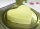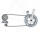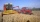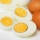# Basic functions - math word problems

1. OrchardOne-eighth of the trees in the fruit plant in winter froze and one-twelfth of damaged disease and pests. Healthy trees remained 152. Is it enough to supply 35 trees to restore the original number of trees in the orchard?
2. SeamstressThe seamstress cut the fabric into 3 parts. The first part was the eighth fabric, the second part was three-fifths of the fabric and the third part had a length of 66 cm. Calculate the original length of the fabric.
3. Average ageThe company of five people has an average age of 46 years. The average age of the first four is 43 years. How many years has the fifth member of this company?
4. Sale discountThe product was discounted so that eight products at a new price cost just as five products at an old price. How many percents is the new price lower than the old price?
5. Sugar productionFrom 1 ton of beet, 150 kg of sugar is produced. To clean 1 ton of sugar 450 kg of lime is consumed. Calculate how many kgs of lime is consumed when processing 1 ton of sugar beet?
6. CuboidThe sum of the lengths of the three edges of the cuboid that originate from one vertex is 210 cm. Edge length ratio is 7: 5: 3. Calculate the length of the edges.
7. SaleA camera has a listed price of \$751.98 before tax. If the sales tax rate is 9.25%, find the total cost of the camera with sales tax included.
8. Cube edgesIf the edge length of the cube increases by 50%, how does the volume of this cube increase?
9. Pupil ageI am a pupil of the primary school, I spent 1/4 of my age on the exercise of parents with children, 1/3 on drawing, 1/6 on flute. I did not have a ring for the first three years of my life and never went to two rings at once. How old am I?
10. WithdrawalIf I withdrew 2/5 of my total savings and spent 7/10 of that amount. What fraction do I have in left in my savings?
11. Exponential equationDetermine the value of having y in the expression (3^y): (4^-1)=36.Y is a natural number greater than zero.
12. Two diggersTwo diggers should dig a ditch. If each of them worked just one-third of the time that the other digger needs, they'd dig up a 13/18 ditch together. Find the ratio of the performance of this two diggers.
13. Bicycle gearThe pedal bicycle wheel has 56 teeth and a rear 20 gear tooth. How many times does the bicycle wheel turn when you make 20 turns of the pedal wheel?
14. HarvestersThe first harvester will harvest the grain from the field in 20 hours, the second, the more powerful in 10 hours. How long will take harvest this field by both harvesters, if the second harvester has to be set up first and this takes 2 hours? The first har
15. ZdeněkZdeněk picked up 15 l of water from a 100-liter full-water barrel. Write a fraction of what part of Zdeněk's water he picked.
16. RainfallA rectangular garden of 25m in length and width 20m in width fall 4mm of water. Express by a fraction in basic form what part of the 60-hectolitre tank we would fill with this water.
17. Eggs3400 eggs sliced hens for February and March. We have to calculate how many hens can make 3400 eggs when one hen give 2 eggs a day for 59 days.
18. PIN codePIN on Michael credit card is a four-digit number. Michael told this to his friend: • It is a prime number - that is, a number greater than 1, which is only divisible by number one and by itself. • The first digit is larger than the second. • The second.
19. Four numbersThe first number is 50% second, the second number is 40% third, the third number is 20% of the fourth. The sum is 396. What are the numbers?
20. FractionsThree-quarters of an unknown number are 4/5. What is 5/6 of this unknown number?

Do you have an interesting mathematical word problem that you can't solve it? Enter it, and we can try to solve it.

To this e-mail address, we will reply solution; solved examples are also published here. Please enter the e-mail correctly and check whether you don't have a full mailbox.

Please do not submit problems from current active competitions such as Mathematical Olympiad, correspondence seminars etc...